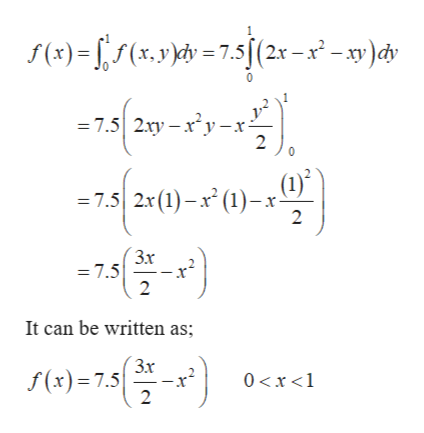# The joint density function of the continuous variables X and Y is fX,Y(x,y) = 7.5x(2-x-y) for 0<X<1 and 0<Y<1.(a) Find the expected value of X+Y.(b) Find fX(x), and fY(y).(c) Find Cov(X,Y).(d) Find Corr(X,Y).

Question
35 views

The joint density function of the continuous variables X and Y is fX,Y(x,y) = 7.5x(2-x-y) for 0<X<1 and 0<Y<1.

(a) Find the expected value of X+Y.

(b) Find fX(x), and fY(y).

(c) Find Cov(X,Y).

(d) Find Corr(X,Y).

check_circle

Step 1

Hello. Since your question has multiple sub-parts, we will solve first three sub-parts for you. If you want remaining sub-parts to be solved, then please resubmit the whole question and specify those sub-parts you want us to solve.

It is given that X and Y are the continuous random variables.

Step 2

a)

The expected value of X+Y, can be calculated as:

Step 3

b)

Function of marginal X can be...help_outlineImage Transcriptionclosef(x)(x.yydv=7.s/(2x-x-)dy = 7.5 2xy-xy-x- 2 (1) = 7.5 2x (1)-x (1) 2 Зх = 7.5 It can be written as; Зх f(x)= 7.5 0 x 1 2 fullscreen

### Want to see the full answer?

See Solution

#### Want to see this answer and more?

Solutions are written by subject experts who are available 24/7. Questions are typically answered within 1 hour.*

See Solution
*Response times may vary by subject and question.
Tagged in

### Other